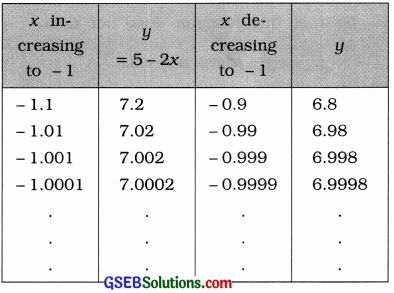# GSEB Solutions Class 12 Statistics Part 2 Chapter 4 Limit Ex 4.2

Gujarat Board Statistics Class 12 GSEB Solutions Part 2 Chapter 4 Limit Ex 4.2 Textbook Exercise Questions and Answers.

## Gujarat Board Textbook Solutions Class 12 Statistics Part 2 Chapter 4 Limit Ex 4.2

Question 1.
Find the values of the following using tabular method:
(1)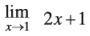Here, f(x) = 2x + 1. Taking the values of x very close to 1, the following table is prepared: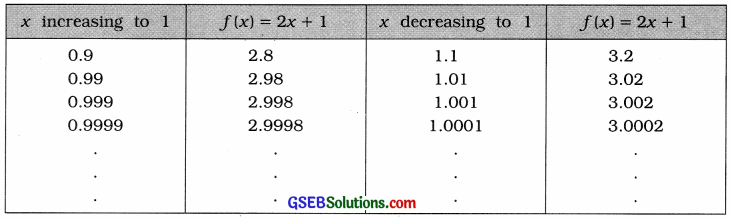It is clear from the table that when x is brought nearer to 1 by increasing or decreasing its value, the value of f(x) approaches to 3. That is, when x → 1, f(x) → 3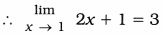(2)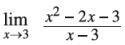Here, f(x) = $$\frac{x^{2}-2 x-3}{x-3}$$
= $$\frac{x^{2}-3 x+x-3}{x-3}$$
= $$\frac{(x+1)(x-3)}{x-3}$$
= x + 1
Taking the values of x very close to 3, the following table is prepared: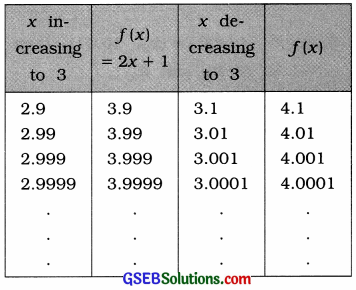It is clear from the table that when x is brought nearer to 3 by increasing or decreasing its value, the value of f(x) approaches to 4. That is, when x → 3, f(x) → 4.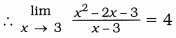(3)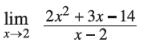Here, f(x) = $$\frac{2 x^{2}+3 x-14}{x-2}$$
= $$\frac{2 x^{2}+7 x-4 x-14}{x-2}$$
= $$\frac{(x-2)(2 x+7)}{x-2}$$
= 2x + 7
Taking the values of x very close to 2, the following table is prepared: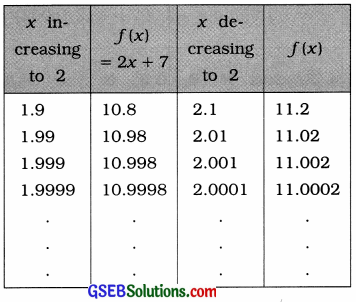It is clear from the table that when x is brought nearer to 2 by increasing or decreasing its value, the value of f(x) approaches to 11. That is, when x → 2, f(x) → 11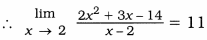(4)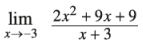Here, f(x) = $$\frac{2 x^{2}+9 x+9}{x+3}$$
= $$\frac{2 x^{2}+6 x+3 x+9}{x+3}$$
= $$\frac{(2 x+3)(x+3)}{x+3}$$
= 2x + 3
Taking the values of x very close to – 3, the following table is prepared: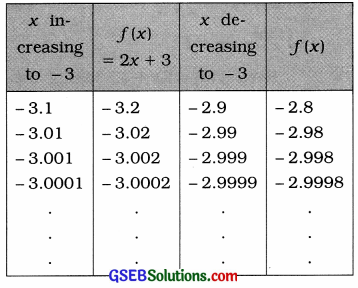It is clear from the table that when x is brought nearer to – 3 by increasing or decreasing its value, the value of f(x) approaches to – 3. That is, when x → – 3, f(x) → – 3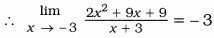(5)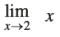Here, f(x) = x. Taking the values of x very close to 2, the following table is prepared: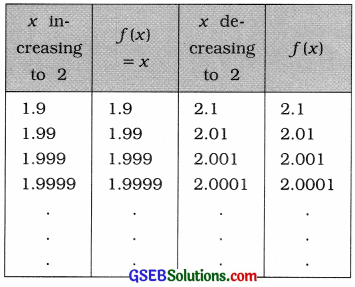It is clear from the table that when x is brought nearer to 2 by increasing or decreasing its value, the value of f(x) approaches to 2. That is, when x → 2, f(x) → 2.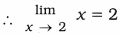Question 2.
Using tabular method, show that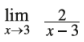does not exist.
Here, f(x) = $$\frac{2}{x-3}$$. Taking the values of x very close to 3. the following table is prepared: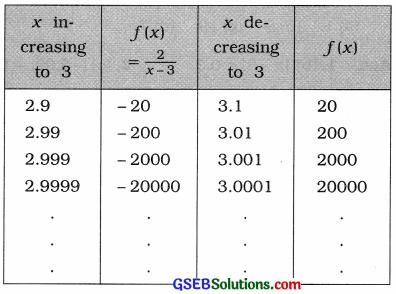It is clear from the table that when x is brought nearer to 3 by increasing or decreasing its value, the value of f(x) does not approaches to a definite value. That is, when x → 3, f(x) does not tend to a definite value. Hence, the limit of this function does not exist.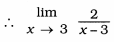does not exist.Question 3.
If y = $$\frac{x^{2}+x-6}{x-2}$$, show that as x → 2, then y → 5 using tabular method.
Here, y = $$\frac{x^{2}+x-6}{x-2}$$
= $$\frac{x^{2}+3 x-2 x-6}{x-2}$$
= $$\frac{(x-2)(x+3)}{x-2}$$
= x + 3
We have to prove that, when x → 2, then y → 5. So we have to find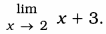Here, y = x + 3. Taking the values of x very close to 2, the following table is prepared :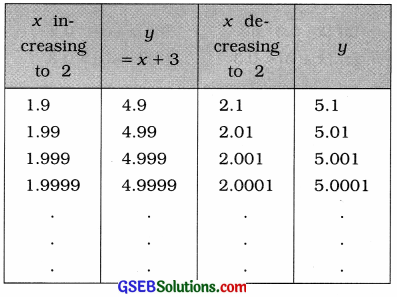It is clear from the table that when x is brought nearer to 2 by increasing or decreasing its value, the value of y approaches to 5. That is, when x → 2, y → 5.
Hence, it is proved that when x → 2, then y → 5.Question 4.
If y = 5 – 2x, show that as x → – 1 then y → 7 using tabular method.